• Category
• >Machine Learning

# How Does K-nearest Neighbor Works In Machine Learning Classification Problem?

• Rohit Dwivedi
• Apr 23, 2020
• Updated on: Jul 05, 2021In a machine learning task, we usually have two kinds of problems that are to be solved either it can be ‘Classification’ or it can be ‘Regression’ problem.

Classification is a process of sorting a given set of data into each different class. Classification can be implemented on both kinds of data structured as well as unstructured. Classes are often referred to as labels or targets which hold different classes. For example, classifying different fruits.

Regression is a problem in which our target holds continuous values or real values. Like prediction of salary or age of a person.

Recommended blog: 7 types of regression techniques in Machine Learning

## K-Nearest Neighbor (KNN)

K-Nearest Neighbor classifier is one of the introductory supervised classifiers, which every data science learner should be aware of. This algorithm was first used for a pattern classification task which was first used by Fix & Hodges in 1951. To be similar the name was given as KNN classifier. KNN aims for pattern recognition tasks.

K-Nearest Neighbor also known as KNN is a supervised learning algorithm that can be used for regression as well as classification problems. Generally, it is used for classification problems in machine learning.

(Must read: Types of learning in machine learning)

KNN works on a principle assuming every data point falling in near to each other is falling in the same class. In other words, it classifies a new data point based on similarity. Let us understand the concept by taking an example:

Example: Two classes green and red and a data point which is to be classified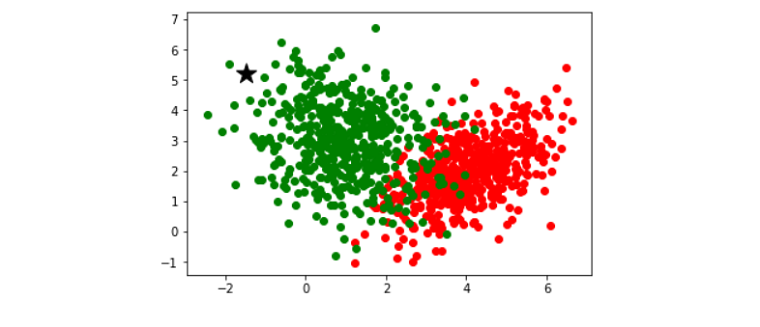Showing a black data point which is classified as of green class

Above is the graph which shows different data points that are red ones, green ones, and a black data point which is classified amongst these two classes.

The above graphs show the same two classes red and green, a black data point which is to be classified by the algorithm either red or green. But how is it computed by the KNN algorithm?

(Similar read: What is K-means Clustering in Machine Learning?)

KNN algorithms decide a number k which is the nearest Neighbor to that data point that is to be classified. If the value of k is 5 it will look for 5 nearest Neighbors to that data point.

In this example, if we assume k=4. KNN finds out about the 4 nearest Neighbors. All the data points near black data points belong to the green class meaning all the neighbours belong to the green class so according to the KNN algorithm, it will belong to this class only. The red class is not considered because red class data points are nowhere close to the black data point.

The simple version of the K-nearest neighbour classifier algorithms is to predict the target label by finding the nearest neighbour class. The closest class to the point which is to be classified is calculated using Euclidean distance.

### Pros of KNN

• A simple algorithm that is easy to understand.

• Used for nonlinear data.

• The versatile algorithm used for both classification as well as regression.

• Gives high accuracy but there are more good algorithms in supervised models.

• The algorithm doesn't demand to build a model, tune several model parameters, or make additional assumptions.

(Referred blog: What is regression analysis?)

### Cons of KNN

• The requirement of high storage.

• Prediction rate slow.

• Stores all the training data.

• The algorithm get slower when the number of examples, predictors or independent variables increases.

•

### Significance of k

Specifically, the KNN algorithm works in the way: find a distance between a query and all examples (variables) of data, select the particular number of examples (say K) nearest to the query, then decide

• the most frequent label if using for the classification based problems,  or

• the averages the label if using for regression-based problems

Therefore, the algorithm hugely depends upon the number of K, such that

• Value of k – bigger the value of k increases confidence in the prediction.

• Decisions may be skewed if k has a very large value.

(Related blog: 6 types of clustering algorithms in ML)

### How to decide the value of k?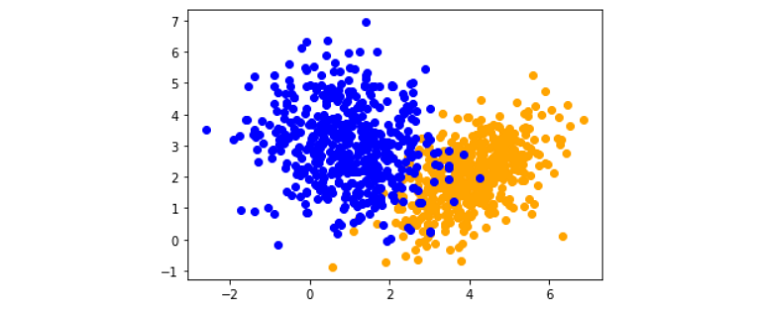Showing two classes orange and blue and X is to be classified amongst these class

Consider the case of two classes one is blue and the other is orange. If we assign the value of k = (1- 4), the X is classified as correctly which is blue class. But if we assign the value of k to be too large then there is misclassification that is orange class.

(Also catch: What is LightGBM Algorithm, How to use it?)

### How to choose K?

• Deciding the k can be the most critical part of K-nearest Neighbors.

• If the value of k is small then noise will have a higher dependency on the result. Overfitting of the model is very high in such cases.

• The bigger the value of K will destroy the principle behind KNN.

• You can find the optimal value of K using cross-validation

## KNN algorithm pseudo code implementation

2. Choose the value of k.

3. For getting the class which is to be predicted, repeat starting from 1 to the total number of training points we have.

4. The next step is to calculate the distance between the data point whose class is to be predicted and all the training data points. Euclidean distance can be used here.

5. Arrange the distances in non-decreasing order.

6. Assume the positive value of k and filtering k lowest values from the sorted list.

7. We have top k top distances.

8. Let ka represent the points that belong to the ath class among k points.

9. If ka>kb then put x in the class.

Let's take a dataset and use the KNN algorithm to get more hands-on experience on how to use KNN for classification. So, we have taken the Iris dataset from the UCI Machine learning Repository.

``````import pandas as pd
print (iris_df.isnull().sum())
print (iris_df.info())
``````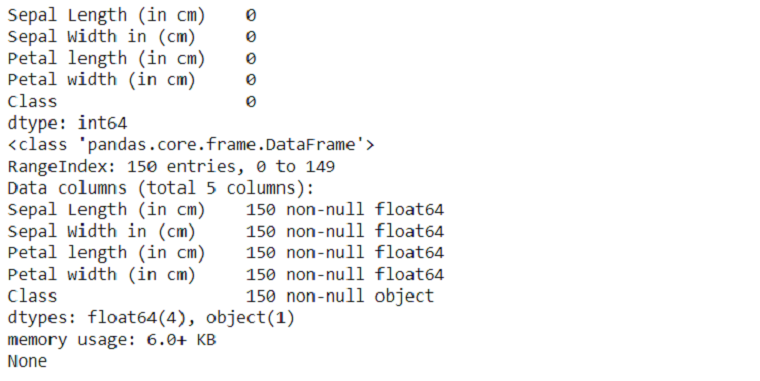Checking null values in the dataset

Initially, I have imported the dataset. Then I have done a bit of EDA, like checking for missing values and information about the dataset. There were no missing values found. All the columns were found to be non-null float64 type and 1 class column to be a non-null object which is our target column.

``````from sklearn.preprocessing import LabelEncoder
LE = LabelEncoder()
iris_df['Class'] = LE.fit_transform(iris_df['Class'])
``````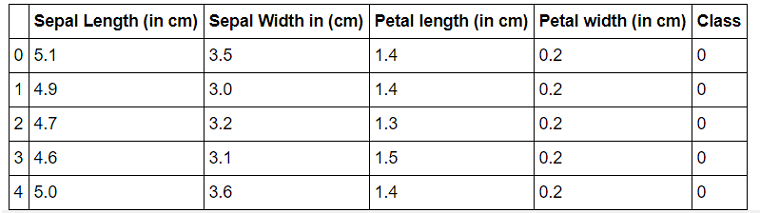Label Encoding in iris Dataset

As you can see the class column is the categorical type and it is needed to label encode the column.

So, I have used LabelEncoder for the same. Label Encoder is a function that gives a label to your categorical columns like in this case it has assigned the values of class that were :

Iris-setosa - 0, Iris-versicolor - 1 , Iris-virginica - 2.

After label encoding, I have assigned our independent features and target features as X & Y respectively. In continuation of that, I have split my data into a 70:30 ratio that is 70% of the training of the model and rest 30% to test the model using train_test_split.

After splitting the data I have imported KNeighborsClassfier from sklearn.

Made an object as NN of KNeighborsClassfier to feed the data to the algorithm. Using NN.fit(X_train,y_train) passed training data.

``````X = iris_df.drop('Class', axis = 1)
y = iris_df['Class']
from sklearn.neighbors import KNeighborsClassifier
from sklearn.model_selection import train_test_split
from sklearn import metrics
test_size = 0.30 # taking 70:30 training and test set
seed = 7  # Random numbmer seeding for reapeatability of the code
X_train, X_test, y_train, y_test = train_test_split(X, y, test_size=test_size, random_state=seed)
NN = KNeighborsClassifier()
NN.fit(X_train,y_train)
``````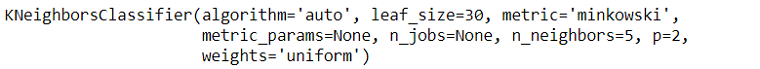K-neighbors Classifier

``````y_pred = NN.predict(X_test)
from sklearn.metrics import accuracy_score
metrics.confusion_matrix(y_test, y_pred)
``````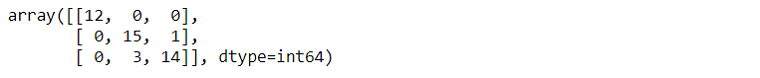``````print(("Test accuracy: ", NN.score(X_test, y_test)))
print(("Train accuracy: ",NN.score(X_train, y_train)))
``````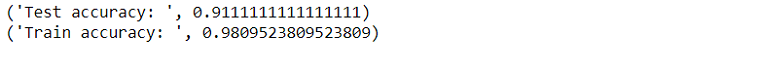Test and training accuracy

After the data got trained predicted classes for X_test that is the rest 30% data using NN.predict(X_test) and stored it into y_pred. Then I have imported accuracy_score from sklearn.metrics to check the accuracy of our model on the test data.

The accuracy score the model gave was around 91%. To check about the evaluation of the model I have used confusion_matrix (Used to evaluate the performance of the model).

The test accuracy was found to be 91% and training accuracy was found to be 98%.

``````from sklearn.model_selection import cross_val_score
print(X_train.shape)
print (int(np.sqrt(X_train.shape)))
maxK = int(np.sqrt(X_train.shape))
print(maxK)
``````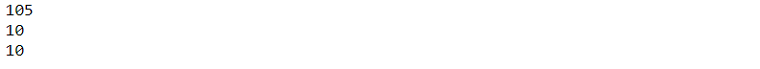Output of cross-validation

Used cross-validation to find the optimal values of k which was found to be 11.

(Suggested blog: How Do Linear And Logistic Regression Work In Machine Learning?)

## Conclusion

In this blog, I have tried to explain the K-Nearest Neighbor algorithm which is used widely for classification. I have discussed the basic approach behind KNN, how it works, metrics used to check about the similarity of data, how to find the optimal value of k and pseudo-code for KNN. Discussed the advantages and disadvantages of using KNN. At last, I have used the KNN algorithm to classify flowers in the iris dataset.

To know more about how to pre-process the data you can visit the link to check different techniques that are used. Also, you can find the jupyter notebook file of the above classification problem here.